Next: In/Out Up: Fluid Section Types: Liquids Previous: Pipe, Gate Valve   Contents

### Pump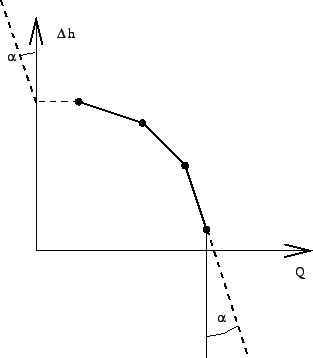A pump is characterized by a total head increase versus total flow curve (Figure 121). The total head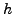is defined by: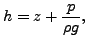(135)

where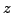is the vertical elevation,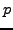is the pressure,is the liquid density and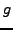is the value of the earth acceleration. The total flow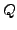satisfies: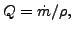(136)

where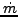is the mass flow. The pump characteristic can be defined underneath a *FLUID SECTION,TYPE=LIQUID PUMP by discrete data points on the curve. The data points should be given in increasing total flow order and the corresponding total head values must be decreasing. No more than 10 pairs are allowed. In between the data points CalculiX performs an interpolation (solid line in Figure 121). For flow values outside the defined range an extrapolation is performed, the form of which depends on the precise location of the flow (dashed lines in Figure 121). For positive flow values inferior to the lowest flow data point, the total head corresponding to this lowest flow data point is taken (horizontal dashed line). For negative flow values the total head sharply increases (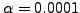) to simulate the zero-flow conditions of the pump in that region. For flow values exceeding the largest flow data point the total head decreases sharply with the same tangent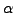.

The gravity acceleration must be specified by a gravity type *DLOAD card defined for the elements at stake. The material characteristiccan be defined by a *DENSITY card.

The liquid is defined by the following parameters (to be specified in that order on the line beneath the *FLUID SECTION, TYPE=LIQUID PUMP card):

• not used
•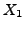•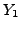•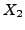•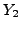• ... (maximum 16 entries per line, use more lines if you want to define more than 7 pairs, maximum 9 pairs in total)

Example files: centheat1.Next: In/Out Up: Fluid Section Types: Liquids Previous: Pipe, Gate Valve   Contents
guido dhondt 2018-12-15